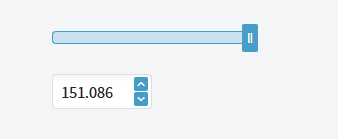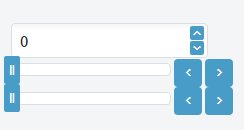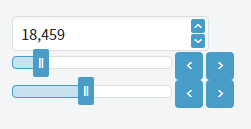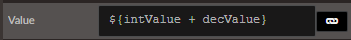# Blazor Slider fine tuning

I am using a slider control in my Blazor application with the Step = .001, Min = 0, Max = 100. I am getting reports from my users that they are unable to properly select the max values using their mouse. When they drag the control selection they can get near the max but almost never hit it. The slider looks amazing and there are no issues with the Step value set to integers. Any ideas?

Hi @mbrown,

Is this correct? If yest this would mean that your slider has 100k steps. This would only work if your Slider is at least 100k pixels wide otherwise more than one step will occupy one pixel.

Here is an example of the max =152 and the Step = .001. Try as hard as I could but I could not set it to the max.Check my reply - this is not a configuration that we can support as one step is a lot less than 1px. Please consider a different input component e.g. Numeric.

Understood much better how the control works with the step/pixel width. If I have the max = non integer value, and the step = integer value is it possible to select the max? ie if max = 153.001 and step = 1 the max I can select is 153. Any suggestions for that?

If the step is more than the fraction yes - you won't be able to select the max value. Similar to how `<input type="range">` behaves.

Well it might not be a good solution but it is something, hear me out.

Have one slider för the number, etc 140. Then have another slider where you se the decimals, etc 0.214.
Then have them added to eachother.

That is an interesting approach. I currently have a slider and numeric input that are in sync with each other such that they have the same min/max/step/change method; when one changes the other reflects that change since the values use binding.

In my focus testing with users they really like the visual and operation of the slider. While the slider does visually look nicer than the numeric input I think I may have to reconsider using it and use the numeric input alone.Just some messing around but you get the idea. Here the slider is very sensetive too and therefor I added buttons which you can change the value with +1 and -1 for the int value and +0.001 and -0.001 for the decimal.

Value of the numeric box is:I just want to mention that a slider should only be used to input discrete values. For example a slider with min = 0, max =2 and step 0.5 can't be used to input 1.3 or 1.6 as a value no matter what you try. If you set the step to 0.1 then your users won't be able to input 1.25 etc.